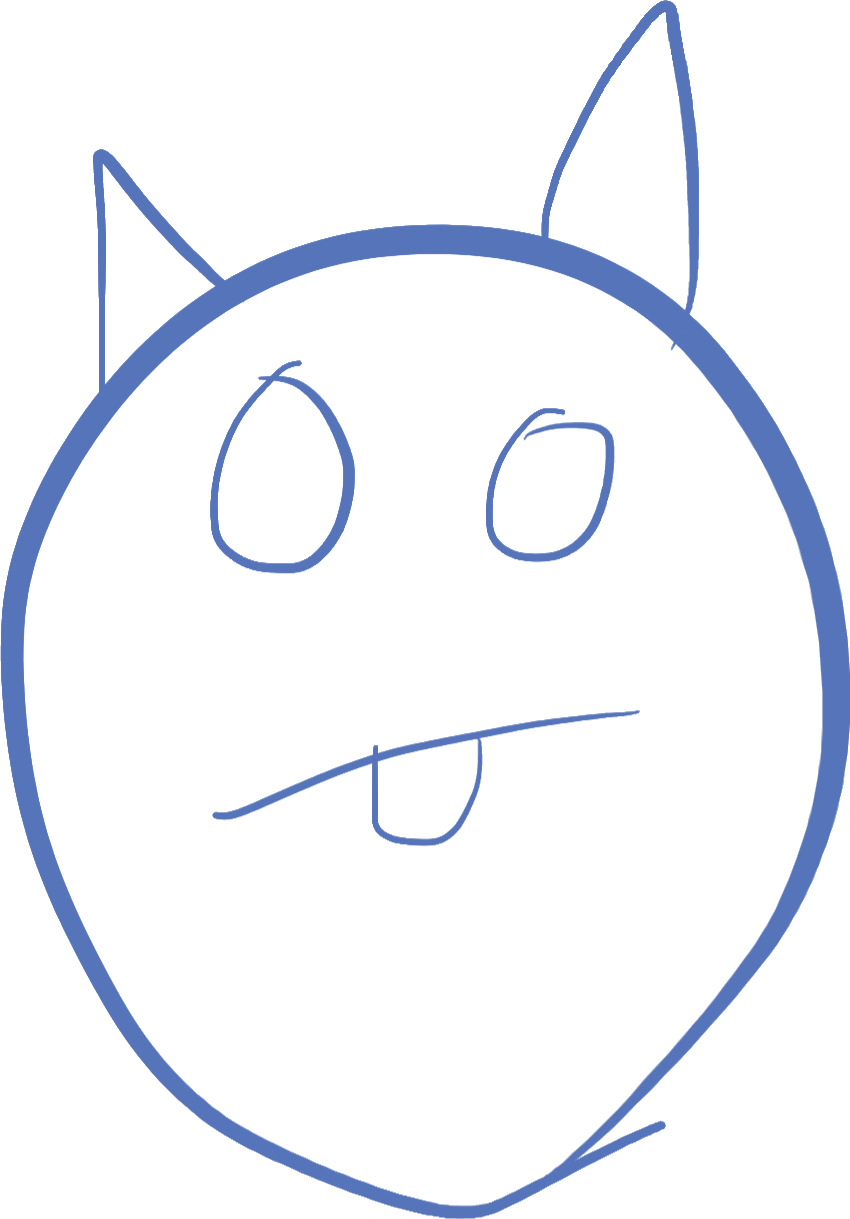# More on collisions and physics

I knew this day would be coming but we have to have a detailed conversation about physics and games. So far, in our games, we have been able to react to the current state of the system. If you watched my explanation video about how the reflection code works, you may have seen, when I changed the trajectory of the ball, so it exited the screen before bouncing, something odd happened. It reflected on the point it was outside the screen. The user would not see that ball, plus it's very unintuitive. Balls don't bounce off of walls by going through them first!

To recap, here is the code from last week that reflects the ball off the walls:

``````if(ball.velocity.y < 0 && ball.pos.y <= ball.radius) {
ball.velocity.y = -ball.velocity.y;
}
if(ball.velocity.x < 0 && ball.pos.x <= ball.radius) {
ball.velocity.x = -ball.velocity.x;
}
if(ball.velocity.y > 0 && ball.pos.y >= GetScreenHeight() - ball.radius) {
ball.velocity.y = -ball.velocity.y;
}
if(ball.velocity.x > 0 && ball.pos.x >= GetScreenWidth() - ball.radius) {
ball.velocity.x = -ball.velocity.x;
}``````

Watch this video below of 2 examples. The first example shows what it looks like when the bounce happens outside the screen, the second shows what it looks like when the bounce happens where it should. I think you would all agree the second looks and feels more correct. Both are from the same ball and trajectory, but in the second, I've taken into account the exact point at which the ball should have hit the wall (including the radius of the ball).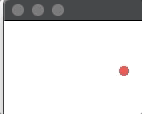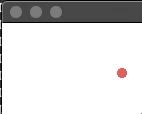A reflection along an axis such as x or y means that we only have to worry about one direction for the math (and thankfully no trigonometry is involved). So for instance, a reflection off of the top of the window which is along the x axis, only the y velocity is reflected, the x velocity is not. We know where the ball would go if it had NOT reflected. So we can correct the position after moving the ball to the place where we detect a reflection should happen, before drawing the next frame.

I'll illustrate this with some images, zoomed in to make the picture easier to see. In both these illustrations, the red ball starts at x = 100, y = 10. The velocity of the ball is (-10, -20), which means for the first frame, the ball moves left by 10 pixels and up by 20 pixels.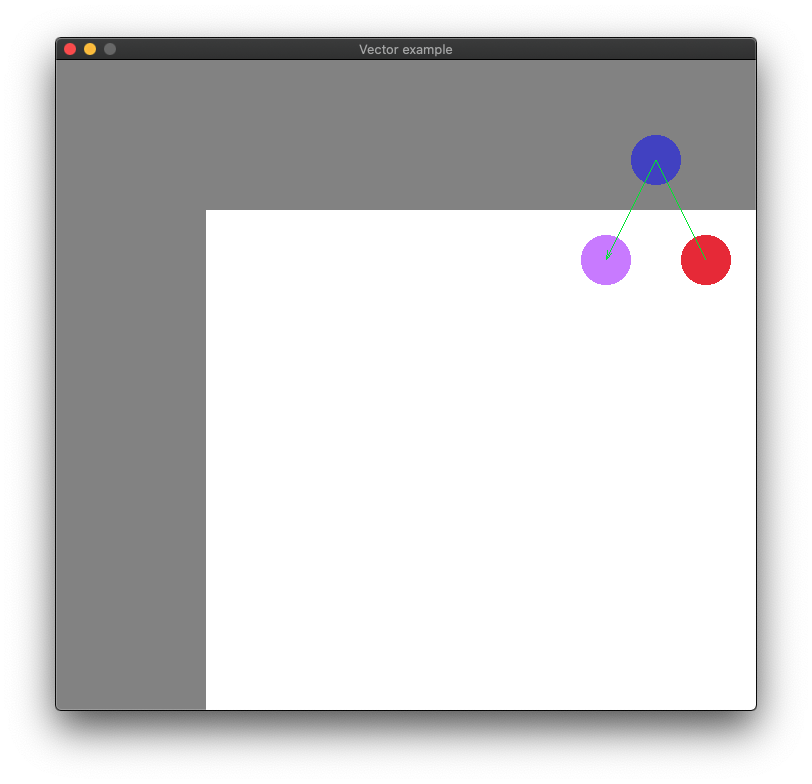This first image shows a blue ball where the code detects a wall collision, at x = 90, y = -10. You can see this is off the screen (the white area is all the user would see, they would not see the gray area). We switch the y velocity to +20, and the next frame (the purple ball) is drawn at x = 80, y = 10. But this isn't what we want, the ball appears to teleport sideways before coming back down (and also disappears for a split second). We really want a reflection when it hits the wall.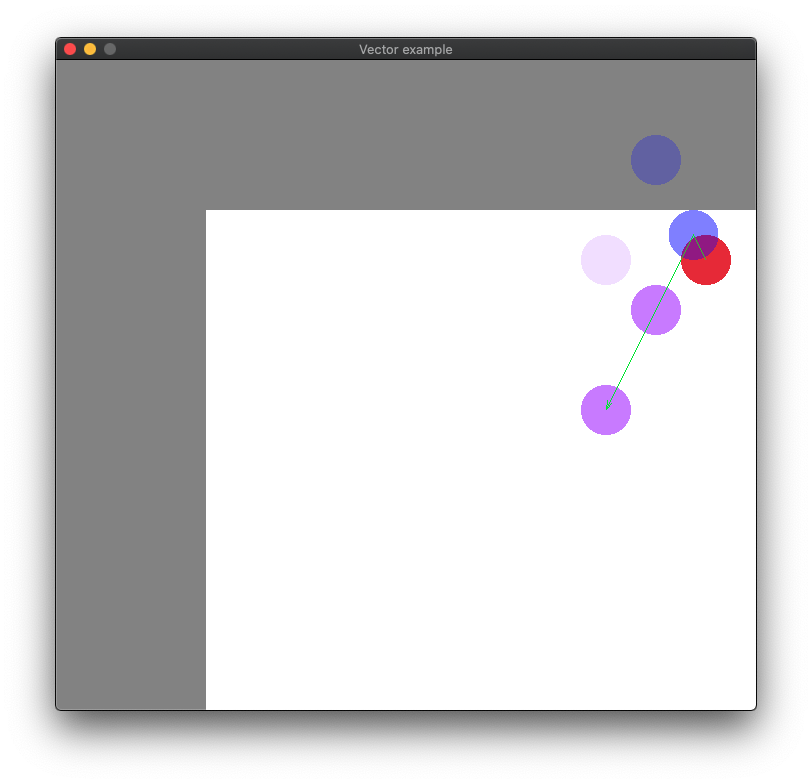The second image shows what we as humans who live in a normal physics world would expect. And that is, a reflection directly off the wall. The faded blue ball touching the wall represents where the reflection would have happened. Note that in the game, this ball isn't drawn because it's between frames. I just drew it here to show you where the bounce would normally happen. The next ball we do draw is the first purple one at x = 90, y = 20. This is because the ball bounced at y = 5 (where the radius of the ball makes the ball touch the wall). The second purple ball is the third frame drawn at x = 80, y = 40 to show the continued path. I also left in an even more faded image of the original ball path so you can see how they line up on the x coordinate.

One thing to realize is that physics simulations are never as exact as real life. We have to consider that our objects only update 60 times a second, and even then, things can happen between those frames. So we have to take shortcuts. The first thing to trigger a change is still the same as before: does the new ball position mean it should have been reflected? That is, is the ball touching or should it have touched the wall? We already have this check, and the velocity adjustment from our current code above:

``````if(ball.velocity.y < 0 && ball.pos.y <= ball.radius) {
ball.velocity.y = -ball.velocity.y;
...``````

But now what we need to do is correct for the difference between where the ball moved and where it should have moved. To do this, we adjust the y position to show that the reflection happened as the user's eyes would expect. To do this against a horizontal or vertical wall (i.e. on an axis) is a simple matter of finding out how far it travelled past the reflection point, retracting that amount, and then adding it to the opposite direction:

``````if(ball.velocity.y < 0 && ball.pos.y <= ball.radius) {
ball.velocity.y = -ball.velocity.y;
float adjustment = ball.radius - ball.pos.y; // ball position when touching the wall, subtracting the current position
ball.pos.y += adjustment + adjustment; // move to the radius position, then move to where it would have reflected
}``````

The `adjustment` variable holds the difference between where it should have bounced, and where it ended up. Think about the math for a second, considering that the `ball.radius` is 5, the `ball.pos.y` is -10. Subtracting -10 from 5 (remember when you subtract a negative number, you are really adding the positive number) yields 15. We add it once to get back to the bounce point (-10 + 15 = 5), and once more to get to where it should have ended up (5 + 15 = 20).

In this way, we can fix all 4 walls for bouncing. In the case of the left wall, the formulas are the same, it's just the x coordinate and not the y coordinate that we need to pay attention to. For the right and bottom walls, the point at which the ball reflects is not 0, but the width or height of the screen. Note that because we are always subtracting where it is from where it should be, we don't have to change the math:

``````if(ball.velocity.y < 0 && ball.pos.y <= ball.radius) { // top wall
ball.velocity.y = -ball.velocity.y;
}
if(ball.velocity.x < 0 && ball.pos.x <= ball.radius) { // left wall
ball.velocity.x = -ball.velocity.x;
}
if(ball.velocity.y > 0 && ball.pos.y >= GetScreenHeight() - ball.radius) { // bottom wall
ball.velocity.y = -ball.velocity.y;
}
if(ball.velocity.x > 0 && ball.pos.x >= GetScreenWidth() - ball.radius) { // right wall
ball.velocity.x = -ball.velocity.x;
}``````

These 4 constructs are very similar, yet each serves a distinct purpose, and can be thought of separately. It's very often in code that you can separate out individual concerns or problems and solve them one at a time. Sometimes you can "factor out" what is happening and write the same code for all the situations. However, in this case, the code is small enough, and each one is different enough, that it would not be worth the effort.

## Homework: make the game playable

For these next 2 weeks, I want you to copy the implementation above to correct odd bounces (this will become important later). But now, we also want to make the game actually a game. The paddle still is doing nothing!

To bounce off of the paddle, we need to reflect not across the bottom wall, but across the top of the paddle. We also need to decide at what point the ball has gone past the paddle such that it cannot be saved. Therefore, the ball should be reflected only if it is touching the paddle top at the instant we check. For now this is enough, because the ball is not travelling too fast. Eventually, we will have to handle the case where the ball bounces off the paddle but never was touching the top line (we will worry about this another day).

To check whether the ball is touching the paddle, we not only have to check against the line that is the top of the paddle, but also that the ball is lined up horiontally with the paddle. This means the ball's horizontal (or x) position cannot be to the left of the the left side of the paddle, or to the right of the right side of the paddle. To do this properly, first check if the ball should be reflected, but is not past the top of the paddle. You will need to check five things:

1. The ball is travelling down (i.e. the y velocity is positive)
2. The ball is low enough that it could be touching the top line of the paddle
3. The ball is not down so far that the TOP of the ball has PASSED the top of the paddle
4. The ball's position horizontally is greater than the left side of the paddle
5. The ball's position horizontally is less than than the right side of the paddle

The first 2 checks are the same as the ones we have above for the bottom wall. Except you can replace the bottom wall position with the paddle's y position (that is its top)

The third check is very similar, but slightly different. You need to check that the ball is not more than `ball.radius` pixels lower than the paddle top.

The fourth and fifth checks are very similar to the left and right wall checks, except we don't have to check the sideways velocity at all, and we do not need to worry about the radius. Just check the positions directly.

If all of these checks are true, then the ball should reflect in it's y velocity. Do not worry in this case about correcting for the appearance of the bounce. We allow this slight error for 2 reasons. First, the ball doesn't go off the screen, so it won't look bad. Second, the paddle isn't always in position to reflect the ball right when it should, you may be frantically moving the paddle over to bounce the ball back, and therefore, it could actually travel below the paddle's top before the paddle is in position. It would look weird to teleport the ball at that point.

I would like you to try to implement these checks on your own, and if you can't please let me know on discord and I'll post some hints. You can try putting all of the checks into one if statement, but you also might want to separate them out into their own if statements (nested inside each other). I would separate out the first 3 that check vertical position from the last 2 that check horizontal.

The code should look similar to this, where you replace the `/* comments */` with actual code

``````if(/*ball moving down and low enough, but not too low*/) {
if(/* ball horizontally lined up with the paddle */) {
/* flip the y velocity of the ball */
}
}``````

This might be more difficult homework than before, but I think you guys can do it! The code should be added after checking wall reflections, but before drawing. As usual, it's always important to update the state of the game and just draw what the state looks like for each frame. Your drawing code shouldn't change at all.

If you get it right, then you can remove the check for the ball hitting the bottom wall, and now you actually have a game that can be played.

Once we have the code that can detect the bouncing off of an object and not just an infinite wall, we have almost all we need to detect collisions with bricks. Next class, we'll hopefully do some work on that and have something that can be not just played but won!